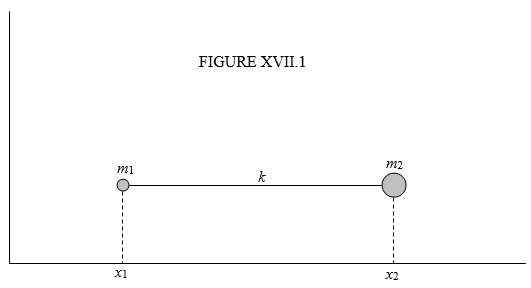$$\require{cancel}$$

# 17.2: The Diatomic Molecule

•• Contributed by Jeremy Tatum
• Emeritus Professor (Physics & Astronomy) at University of Victoria

Two particles, of masses $$m_{1}$$ and $$m_{2}$$ are connected by an elastic spring of force constant $$k$$. What is the period of oscillation?Let’s suppose that the equilibrium separation of the masses – i.e. the natural, unstretched, uncompressed length of the spring – is $$a$$. At some time suppose that the $$x$$-coordinates of the two masses are $$x_{1}$$ and $$x_{2}$$. The extension $$q$$ of the spring from its natural length at that moment is $$q\ =\ x_{2}-x_{1}-a$$. We’ll also suppose that the velocities of the two masses at that instant are $$\dot{x}_{1}$$ and $$\dot{x}_{2}$$. We know from Chapter 13 how to start any calculation in lagrangian mechanics. We do not have to think about it. We always start with $$T$$= ... and $$V$$= ... :

$T=\frac{1}{2}m_{1}\dot{x}_{1}^{2}+\frac{1}{2}m_{2}\dot{x}_{2}^{2}, \label{17.2.1}$

$V=\frac{1}{2}kq^{2}. \label{17.2.2}$

We want to be able to express the equations in terms of the internal coordinate $$q$$. $$V$$ is already expressed in terms of $$q$$. Now we need to express $$T$$(and therefore $$\dot{x}_{1}$$ and $$\dot{x}_{2}$$) in terms of $$q$$. Since $$q\ =\ x_{2}-x_{1}-a$$ we have, by differentiation with respect to time,

$\dot{q}=\dot{x}_{2}-\dot{x}_{1}. \label{17.2.3}$

We need one more equation. The linear momentum is constant and there is no loss in generality in choosing a coordinate system such that the linear momentum is zero:

$0=m_{1}\dot{x}_{1}+m_{2}\dot{x}_{2}. \label{17.2.4}$

From these two equations, we find that

$\dot{x}_{1}=\frac{m_{2}}{m_{1}+m_{2}}\dot{q} \label{17.2.5a}\tag{17.2.5a}$

and

$\dot{x}_{2}=\frac{m_{1}}{m_{1}+m_{2}}\dot{q}. \label{17.2.5b}\tag{17.2.5b}$

Thus we obtain

$T=\frac{1}{2}m\dot{q}^{2} \label{17.2.6}$

and

$$V=\frac{1}{2}kq^{2}$$

where

$m=\frac{m_{1}m_{2}}{m_{1}+m_{2}}. \label{17.2.7}$

Now apply Lagrange’s equation

$\frac{d}{dt}\frac{\partial T}{\partial \dot{q}_{j}}-\frac{\partial T}{\partial q_{j}}=\frac{\partial V}{\partial q_{j}}. \label{13.4.13}\tag{13.4.13}$

to the single coordinate $$q$$ in the fashion to which we became accustomed in Chapter 13, and the equation of motion becomes

$m\ddot{q}=-kq, \label{17.2.8}$

which is simple harmonic motion of period $$2\pi\sqrt{\frac{m}{k}}$$ where $$m$$ is given by Equation $$\ref{17.2.7}$$. The frequency is the reciprocal of this, and the “angular frequency” $$\omega$$, also sometimes called the “pulsatance”, is $$2\pi$$ times the frequency, or $$\sqrt{\frac{k}{m}}$$.

The quantity $$\frac{m_{1}m_{2}}{(m_{1}+m_{2})}$$ is usually called the “reduced mass” and one may wonder is what sense it is “reduced”. I believe the origin of this term may come from an elementary treatment of the Bohr atom of hydrogen, in which one at first assumes that there is an electron moving around an immovable nucleus – i.e. a nucleus of “infinite mass”. One develops formulas for various properties of the atom, such as, for example, the Rydberg constant, which is the energy required to ionize the atom from its ground state. This and similar formulas include the mass $$m$$ of the electron. Later, in a more sophisticated model, one takes account of the finite mass of the nucleus, with nucleus and electron moving around their mutual centre of mass. One arrives at the same formula, except that $$m$$ is replaced by $$\frac{mM}{(m+M)}$$, where $$M$$ is the mass of the nucleus. This is slightly less (by about 0.05%) than the mass of the electron, and the idea is that you can do the calculation with a fixed nucleus provided that you use this “reduced mass of the electron” rather than its true mass. Whether this is the appropriate term to use in our present context is debatable, but in practice it is the term almost universally used.

It may also be remarked upon by readers with some familiarity with quantum mechanics that I have named this section “The Diatomic Molecule” – yet I have ignored the quantum mechanical aspects of molecular vibration. This is true – in this series of notes on Classical Mechanics I have adopted an entirely classical treatment. It would be wrong, however, to assume that classical mechanics does not apply to a molecule, or that quantum mechanics would not apply to a system consisting of a cricket ball and a baseball connected by a metal spring. In fact both classical mechanics and quantum mechanics apply to both. The formula derived for the frequency of vibration in terms of the reduced mass and the force constant (“bond strength”) applies as accurately for the molecule as for the cricket ball and baseball. Quantum mechanics, however, predicts that the total energy (the eigenvalue of the hamiltonian operator) can take only certain discrete values, and also that the lowest possible value is not zero. It predicts this not only for the molecule, but also for the cricket ball and baseball – although in the latter case the energy levels are so closely spaced together as to form a quasi continuum, and the zero point vibrational energy is so close to zero as to be unmeasurable. Quantum mechanics makes its effects evident at the molecular level, but this does not mean that it does not apply at macroscopic levels. One might also take note that one is not likely to understand why wave mechanics predicts only discrete energy levels unless one has had a good background in the classical mechanics of waves. In other words, one must not assume that classical mechanics does not apply to microscopic systems, or that quantum mechanics does not apply to macroscopic systems.

Below leaving this section, in case you tried solving this problem by newtonian methods and ran into difficulties, here’s a hint. Keep the centre of mass fixed. When the length of the spring is $$x$$, the lengths of the portions on either side of the centre of mass are $$\frac{m_{2}x}{m_{1}+m_{2}}$$ and $$\frac{m_{1}x}{m_{1}+m_{2}}$$. The force constants of the two portions of the spring are inversely proportional to their lengths. Take it from there.